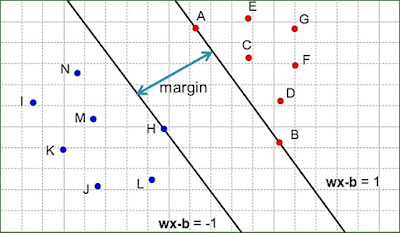# Introduction to Support Vector Machine (SVM)

We would discuss the basics of support vector machines in very clear terms.

We would cover the following 6 topics in this lesson.

### 1. What is a Support Vector Machine?

A support vector machine(svm) also called support vector networks is a supervised learning method that is used for regression, classification and outlier detection.
Given a set of training data set, with each observation marked as belonging to either of two classes, as Support Vector Machine develops a model that assigns a new observation to one class or the other.

### 2. How Does Support Vector Machines Work?

SVM separates the two classes of data using a hyperplane?
But what is a Hyperplane anyway?
Hyperplanes are planes in a lower dimensional space than the given input data that separetes the data into classes. If a space is a 3-dimensional space, then the hyperplanes are 2-dimensionas planes. If a space is 2-dimensional, then its hyperplane are 1-dimensional lines.
Now that you understand what hyperplanes are, let’s continue our discussion on how SVM work.

### 3. The Maximum-Margin HyperPlane

Assuming a linearly separable dataset, we can then select two hyperplanes that seperate the two data classes such that the distance between them is as large as possible. The distance between these two hyperplanes is known as the ‘margin’ or decision boundary for each of the classes. This is illustrated in Figure 2 below.Figure 2: Margin between the two HyperPlanes

The maximum-margin hyperplane is one that lies at the midpoint between these two hyperplanes.

Now the two classes could be defined using the linear model given by:

y(x) = wTφ(x) + b

where φ(x) is a transformation function and
b is the bias

For the example above example in Figure 2, the parameters has to  be chosen such that for the blue data points, y(x) < 0 and for the red data points y(x) > 0.
So we can now define the equations for the two classes as

For the blue class on the left

wTφ(x) + b = -1

For the red class on the right:

wTφ(x) + b = +1

This means that each data point must lie on the correct margin
We can now determine the maximum-margin hyperplane by fitting a line exactly half-way between the two hyperplanes. This is illustrated in Figure 3 below.

• SVMs are very efficient in handling data in high-dimensional space
• It is memory efficient because it uses a subset of training points in the support vectors
• It is very effective when the number of dimensions is greater than the number of observations

### 5. Cons of SVM

• It is not suitable for very large data sets
• It is not very efficient for data set have much outliers
• It doen’t directly provide an indication of probability estimation

### 6. Introduction to the Kernel Trick

When it comes to solving complex classification function e.g for very large data sets, then the simple SVM method may not be very effective. A method known as the kernel trick is employed.
A function that takes as input, vectors in the original input space and returns the dot product of the vectors in the feature space is called a Kernel Function or the Kernel Trick. We would not discuss more on the kernel trick here since there is another complete lesson on the Kernel Trick

### 7. Final Notes

We have examined the basics of SVM or Introduction to Support Vector Machines. This lesson is meant to give you an overview of what the concept is. For indepth study of SVM, I would recommend getting a  textbook such as ‘Pattern Recognition and Machine Learning‘ by Bishop.

I would like to thank you for reading. For any observation, you can leave a comment on the form on the left side of the page.